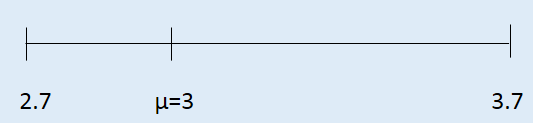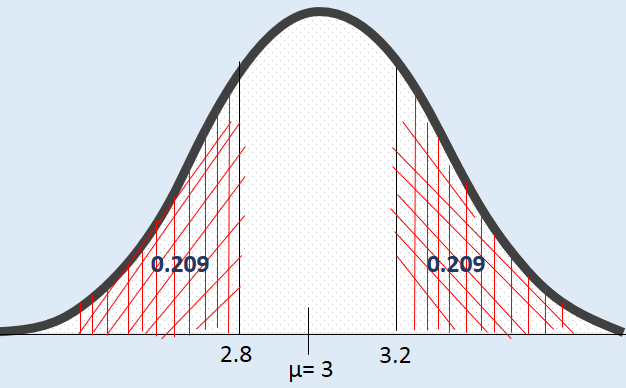### Introduction to Hypothesis Testing

#### Hypothesis:

A hypothesis is an assumption about a population parameter. It is a statement about the population that may or may not be true. Hypothesis testing aims to make a statistical conclusion about accepting or not accepting the hypothesis.

So, a statistical hypothesis is an assertion or conjecture concerning one or more populations. To prove that a hypothesis is true, or false, with absolute certainty, we would need absolute knowledge. That is, we would have to examine the entire population. Instead, hypothesis testing concerns on how to use a random sample to judge if it is evidence that supports or not the hypothesis.

Hypothesis testing is formulated in terms of two hypotheses:

• H0: the null hypothesis;
• HA: the alternate hypothesis

The null hypothesis (H0) often represents either a skeptical perspective or a claim to be tested. The alternative hypothesis (HA) represents an alternative claim under consideration and is often represented by a range of possible parameter values.

The skeptic will not reject the null hypothesis (H0), unless the evidence in favor of the alternative hypothesis (HA) is so strong that she rejects H0 in favor of HA.

#### Null Hypothesis (H0):

• Represents the status quo.
• The hypothesis that states there is no statistical significance between two variables in the hypothesis.
• Believed to be true unless there is overwhelming evidence to the contrary.
• It is the hypothesis the researcher is trying to disprove.

Example:

It is hypothesised that flowers watered with lemonade will grow faster than flowers watered with plain water.

Null hypothesis:

There is no statistically significant relationship between the type of water used and the growth of the flowers.

#### Alternative Hypothesis (HA):

• Inverse of the null hypothesis.
• States that there is a statistical significance between two variables.
• Holds true if the null hypothesis is rejected.
• Usually what the researcher thinks is true and is testing

Null hypothesis:

If one plant is fed lemonade for one month and another is fed plain water, there will be no difference in growth between the two plants.

Alternative Hypothesis:

If one plant is fed lemonade for one month and another is fed plain water, the plant that is fed lemonade will grow more than the plant that is fed plain water

In hypothesis testing, we want to test is if HA is “likely” true. So, there are two possible outcomes:

• Reject H0 and accept HA because of sufficient evidence in the sample in favor or HA;
• Do not reject H0 because of insufficient evidence to support HA.

#### Note:

Failure to reject H0 does not mean the null hypothesis is true. There is no formal outcome that says “accept H0.” It only means that we do not have sufficient evidence to support HA.

#### Example:

In a jury trial the hypotheses are:

• H0: defendant is innocent;
• HA: defendant is guilty.

H0 (innocent) is rejected if Ha (guilty) is supported by evidence beyond “reasonable doubt.” Failure to reject H0 (prove guilty) does not imply innocence, only that the evidence is insufficient to reject it.

#### Hypothesis Testing via CI:

Earlier we calculated a 95% confidence interval for the average number of exclusive relationships college students have been in to be (2.7, 3.7). Based on this confidence interval, do these data support hypothesis that college students on average have been in more than 3 exclusive relationship.

Then our NULL Hypothesis will be will like this:

H0: µ = 3 College students have been in 3 exclusive relationships, on average.

HA: µ > 3 College students have been in more than 3 exclusive relationships, on average.

For this case, our intervals span from 2.7 to 3.7 and the null value µ=3 are actually included in the interval. And the interval says any value within this range could conceivably be the true population mean therefore we cannot reject the null hypothesis in favor of the alternative.This is quick and dirty approach for hypothesis testing. However, it doesn’t tell us the likelihood of certain outcome under the null hypothesis. In the other words it does not tell us  the p value.

Note:

We always do hypothesis testing for population parameters. We never hypothesized sample statistics.

#### Hypothesis testing via p-Value:

The p-value is a way of quantifying the strength of the evidence against the null hypothesis and in favor of the alternative. Formally the p-value is a conditional probability

###### p-value:

The p-value is the probability of observing data at least as favorable to the alternative hypothesis as our current data set, if the null hypothesis is true. We typically use a summary statistic of the data, here the sample mean, to help compute the p-value and evaluate the hypotheses.

If we calculate the p-value for the same problem discussed in confidence interval section (Example 2).

P (Observed or more extreme outcome | H0 true) =?

When

N=50

¯x = 3.2

S= 1.74

SE== 1.74/SQRT (50) = 0.246

We are trying to find the value of P (¯x > 3.2 | H0: µ= 3) which is coming from null hypothesis.

Since we are assuming null hypothesis to be true, we can use that to construct the sampling distribution based on the Central Limit Theorem.

¯x ~ N (µ= 3, SE= 0.246) Here 3 is coming from null hypothesis as we are assuming null hypothesis is true. See the below picture, our area of interest for p-value is the red shaded area.The Z-Score can be calculated by this formula

Test statistics, Z= (3.2-3)/0.246 = 0.81

p-value = P(Z > 0.81) = 0.209

• We use the test statistic to calculate the p-value, the probability of observing data at least as favorable to the alternative hypothesis as our current data, if the null hypothesis was true.
• If the p-value is low (lower than the significance level, α, which is usually 5 %) we say that it would be very unlikely to observe the data if the null hypothesis were true, and hence reject H0.
• If the p-value is high (higher than α) we say that it is likely to observe the data even if the null hypothesis were true, and hence do not reject H0.

Since p-value for this case is 0.209 and it is higher than 0.05, so we do not reject the null hypothesis.

What is that meaning context of this question? Our null hypothesis was that college student on average have 3 exclusive relationships vs the alternative hypothesis was college students have been in more than 3 exclusive relationships, on average. In this case, we fail to reject null hypothesis as we do not have enough evidence to reject null hypothesis. That sets the population average of number of exclusive relationships college student have been in to 3.

###### Interpreting the p-value:

IF in fact college students have been in 3 exclusive relationships on average, there is a 21% chance that a random sample of 50 college students would yield a sample mean of 3.2 or higher. This is a pretty high probability, so we think that  a sample mean of 3.2 or more exclusive relationships is likely to happen simply by chance.

###### How we made this decision?
• Since p-value is high(higher than 5%) we fail to reject H0.
• These data do not provide convincing evidence that college students have been in more than 3 relationship on average.
• The difference between the null value of 3 relationships and the observed sample mean of 32 relationship is due to chance or sampling variability.

#### Two-sided hypothesis testing with p-values:

Often instead of looking for a divergence from the null in a specific direction, we might be interested in divergence in any direction. We call such hypothesis tests two-sided (or two-tailed). The definition of a p-value is the same regardless of doing a one or two-sided test, however the calculation is slightly different since we need to consider “at least as extreme as the observed outcome” in both direction away from the mean.

For the above example if we want to do the two-sided hypothesis testing then we have to find P (¯x > 3.2 OR (¯x < 2.8 | H0: µ= 3).

How to we come up with 2.8?

As, 3.2-3=0.2

so, 3-0.2=2.8p-value =  P( Z > 0.81) + P( Z < -0.81)

= 2*0.209

=0.418